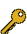Last Update: 2023-04-01 06:39:18

## 8-c. \$BJ,N%!&Cj=P(B

\$B:G=*99?7F|;~!'(B2021-08-21 18:44:01\$B\$3\$NJ,N`\$G\$h\$/;H\$o\$l(B
\$B\$F\$\$\$k%-!<%o!<%I(B
\$B%-!<%o!<%I(B\$B
supercritical CO2 extraction1\$B7o(BCO2-expanded hexane1\$B7o(Bcarbon dioxide1\$B7o(BPCL nanosuspension1\$B7o(Bbio-oil1\$B7o(BHydrothermal1\$B7o(BJapanese essential oil1\$B7o(Bsupercritical carbon dioxide1\$B7o(BExtraction of coffee1\$B7o(Blow pressure1\$B7o(Benzyme assisted1\$B7o(Bextraction of emulsion1\$B7o(BExtraction1\$B7o(BExtraction of strawberry leaf1\$B7o(BMonocyclic compounds1\$B7o(B\$B\$BHV9f(B\$B9V1iBjL\!?H/I=\$B%-!<%o!<%I(B\$BH/I=7A<0(B
26\$B9b299b05?e\$rMQ\$\$\$?%3!<%R!<@8F&Cj=P\$K\$*\$1\$kC14D@8@.J*\$N5sF0(B
(\$B1'ET5\Bg1!CO(B) \$B!{(B(\$B3X(B)\$BCSC+(B \$B2BO/(B\$B!&(B (\$B1'ET5\Bg9)(B) (\$B@5(B)\$B:4F#(B \$B9d;K(B\$B!&(B (\$B@5(B)\$B0KF#(B \$BD>\$B!&(B (\$B1'ET5\BgG@(B) \$BFsIS(B \$B8-0l(B
Extraction of coffee
Hydrothermal
Monocyclic compounds
P
88\$BFs;@2=C:AGKDD%1UBN\$K\$h\$kJF9G\$+\$i\$N%P%\$%*%*%\$%k\$NCj=P(B
(\$B@EBg1!9)(B) \$B!{(B(\$B3X(B)\$B0KF#(B \$B3+CN(B\$B!&(B (\$B@EBg9)(B) \$B@>K\(B \$BM'N\$(B\$B!&(B (\$B@EBg1!9)(B) Le Thi Thien Ly\$B!&(B (\$B@5(B)\$B9&(B \$B>;0l(B\$B!&(B (\$B@5(B)\$B2,Eg(B \$B\$\$\$E\$_(B\$B!&(B (\$B@5(B)\$B:48E(B \$BLT(B
CO2-expanded hexane
Extraction
bio-oil
P
98\$BFs;@2=C:AG(B-\$B?e:.9gMOG^\$K\$h\$k%\$%A%4MUCj=P\$K\$*\$1\$k05NO8z2L(B
(\$B1'ET5\Bg1!CO(B) \$B!{(B(\$B3X(B)\$B9S4,(B \$B;V7n(B\$B!&(B (\$B3X(B)\$BCSC+(B \$B2BO/(B\$B!&(B (\$B1'ET5\Bg9)(B/\$B1'ET5\Bg%m8&(B) (\$B@5(B)\$B:4F#(B \$B9d;K(B\$B!&(B (\$B1'ET5\BgG@(B/\$B1'ET5\Bg%m8&(B) \$B9uAR(B \$B7r(B\$B!&(B (\$B1'ET5\Bg9)(B) (\$B@5(B)\$B0KF#(B \$BD>
Extraction of strawberry leaf
carbon dioxide
low pressure
P
180\$BD6NW3&%(%^%k%7%g%sCj=P\$K\$h\$k(Bpolycaprolactone\$B%J%N7|By1U\$N@8@.(B
(\$BEl9)BgJ*(\$B3X(B)Bao Quiqi\$B!&(B (\$B@5(B)\$B?%ED(B \$B9LI'(B\$B!&(B (\$B@5(B)\$B2<;3(B \$BM52p(B
supercritical carbon dioxide
extraction of emulsion
PCL nanosuspension
P
617\$BD6NW3&Fs;@2=C:AG\$K\$h\$k%f%:\$NOB@:L}Cj=P\$K\$*\$1\$k9ZAGA0=hM}\$*\$h\$S4%Ag
(\$BElKLBg1!9)(B) \$B!{(B(\$B3X(B·\$B5;4p(B)\$B>.NS(B \$BBsLp(B\$B!&(B (\$BElKLBg9)(B) \$B:4!9LZ(B \$BF7H~(B\$B!&(B (\$BElKLBg1!9)(B) (\$B@5(B)\$BLZ2<(B \$BKS(B\$B!&(B (\$B@5(B)\$BJ?2l(B \$BM\$Li(B\$B!&(B (\$B@5(B)\$BEOn5(B \$B8-(B
supercritical CO2 extraction
enzyme assisted
Japanese essential oil
P

\$B9V1i?=\$79~\$_0lMw(B(\$B%7%s%]%8%&%`HV9f!&9V1iJ,N`HV9fJL(B)

\$B

This page was generated byeasp 2.46; update.pl 2.37 (C)1999-2015 kawase